Trigonometry Tutorial (Part 3)

Solving Oblique Triangles

Without Using the Law of Sines or the Law of Cosines

For solving oblique triangles by using the Law of Sines and the Law of Cosines, click here.

An oblique triangle is one which contains no right angles.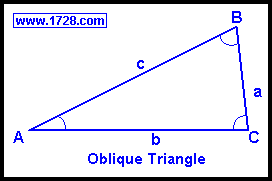So, what's the point in showing another method when we just learned how to do this in Part 2? For one thing, it demonstrates how important it is to know the fundamental mathematical concepts of the Pythagorean Theorem, triangle area formulas, and so on. Also, if you are taking a test and you forget exactly how the Law of Sines or the Law of Cosines works, you can always find a solution by going to more basic concepts. These solutions solve all of the problems presented in Part 2 except that the Law of Sines and the Law of Cosines were not used. If you compare these solutions to those in Part 2, you will see they don't require that much more work.

Two Angles and an Included Side

Example
A triangle has 2 angles of 75° and 25° and the included side is 5. What is the other angle and the other 2 sides?
Let's call Angle A = 25°, Angle B = 75° and side c = 5.
Angle C is easily found by: ∠C = 180° - ∠A - ∠B     = 180° - 25° - 75°
∠C = 80°
Dropping a perpendicular 'hgt' (for 'height') from Angle C to side 'c', splits triangle ABC into 2 right triangles.
So, angle BCD =180° - 90° - ∠B     =15°
and Angle ACD =180° - 90° - ∠A     =65°
Using this information and a trigonometry calculator, we can find the values of the 2 parts of line 'c'. (see diagram below).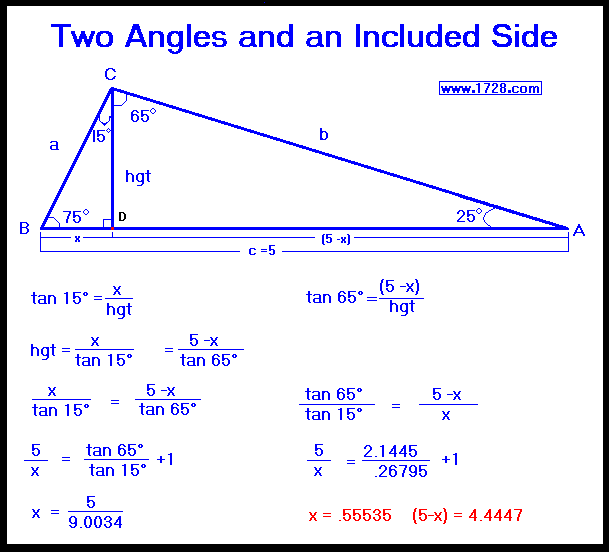Now, the remaining calculations are quite straightforward.
side a = .55535 ÷ sine(15°)   side a = .55535 ÷ .25882
side a = 2.1457
side b = 4.4447 ÷ sine(65°)   side b = 4.4447 ÷ .90631
side b = 4.9042

Two Sides and a Non-Included Angle

When solving for a triangle with 2 known sides and a non-included angle, there may be no solution, one solution or even two solutions.
In a triangle of sides 'c', 'a' and its opposite Angle 'A', the number of solutions is calculated by:
• If a < c•sin(A) no solution
• If a = c•sin(A) one solution: ∠C=90° ∠B=(90°-∠A) and side b=(c•cos(A))
• If c>a>c•sin(A) two solutions.
• If a>=c one solution
Example
A triangle has sides of 7 and 8 and a non-included angle of 60°
What are the other angles and the other side?
Let's say side a=7, c=8 and Angle A=60°
Dropping a perpendicular 'hgt' from Angle B, we find its length by:
hgt = sine 60° • 8     = .86603 • 8 =   6.9282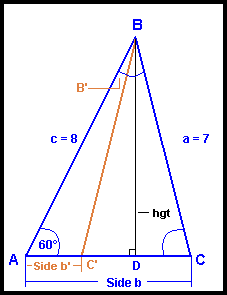Angle C = arc sine (hgt ÷ side a) = arc sine (6.9282 ÷ 7) = arc sine (.98974)
Angle C = 81.785°
Angle B is easily found by: ∠B= 180° -60° - 81.785°
Angle B = 38.215°

Side b = AD + DC
To solve these, we'll use the Pythagorean Theorem:
Side AD² = c² - hgt²   = 8² - 6.9282²   Side AD=4
Side DC² = a² - hgt²   = 7² - 6.9282²   Side DC=1
Side b = AD + DC = 4 +1   Side b = 5

Is there a second solution?
If c>a>c•sin(A) there are 2 solutions.

8 > 7 > (8 • sin (60°))
Since 8 > 7 > 6.92824, there are two solutions.

Whenever there are two solutions, ∠C and ∠B will each have 2 values.
We will designate the "second Angle C" as C' and we find it by:
∠C' = 180° - ∠C     ∠C' = 180° - 81.789°
∠C' = 98.213°
We find ∠B' by:
∠B' = 180° -∠A - ∠C'     ∠B' = 180° -60° - 98.213°
∠B' = 21.787°

Since side b = AD + DC, then side b' = AD - DC = 4 - 1
Side b' = 3

Two Sides and the Included Angle

Example
A triangle has sides of 4 and 9 with an included angle of 30°. What are the other 2 angles and the length of the other side?
Let's say side a=4 and side b=9 and angle C=30°
Dropping a perpendicular 'hgt' from Angle B to side 'b', splits triangle ABC into 2 right triangles and splits side b into 'x' and '9-x'.
So, angle CBD =180° - 90° - ∠C     = 60°
hgt= sine 30° • 4 = .5 • 4     hgt = 2
Line segment 'x' = sine 60° • 4   'x' = .86603 • 4     'x' = 3.4641
Line DA (or 9-x) = 9 - 3.4641 = 5.5359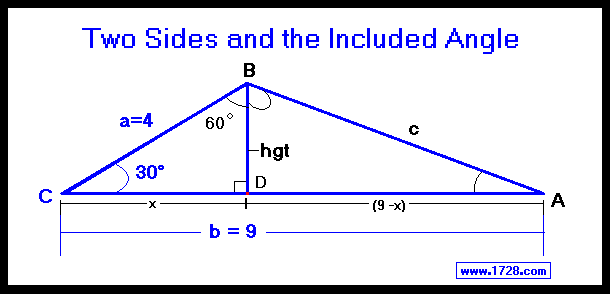Arc tangent (A) = 2 ÷ 5.5359   = .36128   Arctan(A) = .36128
∠A = 19.864°
Arc tangent (DBA) = 5.5359 ÷ 2 = 2.7680   Angle (DBA) = 70.136°
Angle B is found by adding CBD and ABD which equals 60° + 70.136°
∠B = 130.136°
(We could have found Angle B just as easily by ∠B = 180° - ∠A - ∠C)
Side c = 2 ÷ Sine 19.864° = 2 ÷ .33979   = 5.886
Side c = 5.886
(We also could have found side 'c' by using the Pythagorean Theorem).

All Three Sides Of A Triangle

EXAMPLE:
A triangle has sides of length four, five and six. What are all 3 angles?
Let's say that side a=4, side b=5 and side c=6.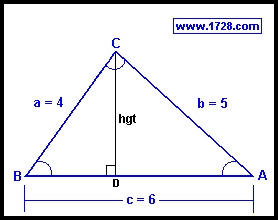Yes, this could be solved in many different ways, but here's a "neat" solution.
Remember Heron's formula from the triangle page? It states that
triangle area = Square Root (s•(s-a)•(s-b)•(s-c))

where 's' is the semi-perimeter of the triangle.

s=(4 + 5 + 6) / 2       s= 7.5

area = Square Root (7.5 •(7.5 -4)•(7.5 -5)•(7.5 -6))
area = Square Root (98.4375)
area = 9.9216
So, how does this help solve the triangle? Well, there is another area formula:
triangle area = ½ • base • height and therefore,
height = (2 • area ÷ base)
height = (2 • 9.9216 ÷ 6)
height = 3.3072
∠B = arc sine (hgt ÷ side a)
∠B = arc sine (3.3072 ÷ 4)
∠B = arc sine (.8268)
∠B = 55.771°
∠A = arc sine (hgt ÷ side b)
∠A = arc sine (3.3072 ÷ 5)
∠A = arc sine (.66144)
∠A = 41.41°
We then easily solve Angle C using this equation:
Angle C   =   180°   -55.771°   - 41.41°
∠C = 82.819°

Go To Page One
Basic Trigonometric Functions

Go To Page Two
Solving Oblique Triangles Using Law of Sines and Law of Cosines